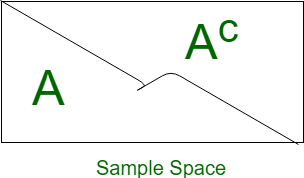# GRE Data Analysis | Probability

Probability is a numerical representation of the chance of occurrence of a particular event. Here the event is the word used to describe any particular set of the outcome.For Example, when a coin is tossed the total number of possible outcomes are 2 i.e {Head, Tail}. And the probability of getting head, P(Head) is 1/2. since the number of success for getting head is 1.

Important Terminology:

• Sample space:
Total number of possible outcome for a event is know as its sample space. It is generally denoted by S.
• Equally likely:
Two events are equally likely if they have equal probability of occurrence.
• Event:
Event can also be described as the subset of sample space.
• Trail:
It is an action which results in one or many outcomes.
• Mutually Exclusive:
Two events are said to be mutually exclusive when both can not occur at same time.
• Independent Event:
Two events lets say A and B, are independent of each other when occurrence of one does not effect occurrence of other. When two events, A and B, are independent, the probability of both occurring is:

P(A and B) or P(A ∩ B) = P(A)·P(B)
Axioms of Probability:

1. 0 ≥ P(E) ≤ 1, i.e Probability of an event is always greater than or equal to zero and less than or equal to one.
2. P(S) = 1, Here S represents sample space. The probability of occurrence of atleast one event of sample space is 1. If the probability of event is 1 then it is certain that the event will occur.
3. P(A ∪ B) = P(A) + P(B), where A and B are two mutually exclusive events, i.e P(A ∩ B) = φ
Note:

1. P(Ac)= 1 – P(A), where P(Ac) is complement of P(A).2. When two events, A and B, are not-mutually exclusive, the probability that A or B is:
P(A or B) = P(A) + P(B) - P(A and B)

Example-1: Tokens numbered 1 to 20 are mixed up and then a token is drawn at random. What is the probability that the token drawn has a number which is a multiple of 3 or 5?
Solution: Total possible outcome,

S = {1, 2, 3, 4, 5, ...20}

The favorable outcome, which is multiple of 3 or 5 is

E = {3, 6, 9, 12, 15, 18, 5, 10, 20}

Then,

P(E) = n(E)/ n(S)

where n(E) represents number of favorable outcome

P(E) = 9/20

Example-2: An Urn contains 2 red, 3 green, and 5 blue balls. Two balls are drawn at random. What is the probability that none of the balls drawn is blue?
Solution:

Total number of ball = 10, Number of blue ball = 5
P(Blue) = Number of blue balls / total number of balls
P(Blue) =  5/10 = 1/2

P(not blue) = 1 - P(Blue)
P(not blue) = 1- 1/2 = 1/2 

Example-3: Find the probability of getting sum more that 7, when we simultaneously throw a pair of dice.
Solution: Total number of outcome,

= 62 = 36

Number of favorable outcome,

= {(2, 6)
(3, 5) (3, 6)
(4, 4) (4, 5) (4, 6)
(5, 3) (5, 4), (5, 5) (5, 6)
(6, 2) (6, 3) (6, 4) (6, 5) (6, 6)} = 15

P(sum more than 7) = 15/36 = 5/12 

Example-4: Find the probability of getting two consecutive Heads when three coins are tossed simultaneously.
Solution:

Total number of outcome,
= 23 = 8

These are,
{(HHH), (HTH), (HHT), (HTT), (THH), (THT), (TTH), (TTT)}

Number of favorable outcome,
= {(HHH), (HHT), (THH)} = 3

P(getting two consecutive heads) = 3/8  

My Personal Notes arrow_drop_upCheck out this Author's contributed articles.

If you like GeeksforGeeks and would like to contribute, you can also write an article using contribute.geeksforgeeks.org or mail your article to contribute@geeksforgeeks.org. See your article appearing on the GeeksforGeeks main page and help other Geeks.

Please Improve this article if you find anything incorrect by clicking on the "Improve Article" button below.

Article Tags :

Be the First to upvote.

Please write to us at contribute@geeksforgeeks.org to report any issue with the above content.